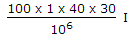# Electronics and Communication Engineering - Measurements and Instrumentation

### Exercise :: Measurements and Instrumentation - Section 1

21.

The Lissajous pattern observed on screen of CRO is a straight line inclined at 45° to x axis. If X-plate input is 2 sin ωt, the Y-plate input is

 A. 2 sin ωt B. 2 sin (ωt + 45°) C. 2 sin (ωt - 45°) D. 22 sin ωt + 45°)

Explanation:

When signals to X and Y plates are in phase, the display is a straight line inclined at 45°.

22.

The angle δ for a mica capacitor is about

 A. 25° B. 15° C. 5° D. 1°

Explanation:

Mica capacitor has very low loss and loss angle δ is very very small.

23.

A digital voltmeter has a read out range from 0 to 999 counts. If the full scale reading is 9.999 V, the resolution is

 A. 1 V B. 0.01 V C. 1 mV D. 1 μV

Explanation:

Resolution is the smallest change in the quantity being measured which will cause an observable change in output. In this case it is 1 mV.

24.

The coil of a moving coil meter has 100 turns, is 40 mm long and 30 mm wide. The control torque is 240 x 10-6 N-m on full scale. If B = 1 Wb/m2, range of meter is

 A. 1 mA B. 2 mA C. 3 mA D. 4 mA

Explanation:

NBAI = Controlling torque or= 240 x 10-6 or I = 2 mA.

25.

Consider the following statements: In a differential amplifier using JFET, the differential output offset is due to

1. mismatch between FET parameters
2. difference in values of resistances used in circuit
3. variation in supply voltage.
Of the statements, the correct statements are

 A. 1 and 2 B. 1, 2, 3 C. 2, 3 D. 1, 3